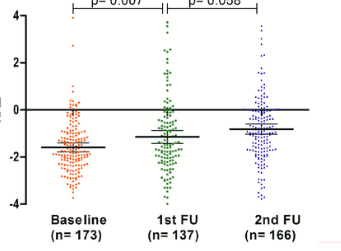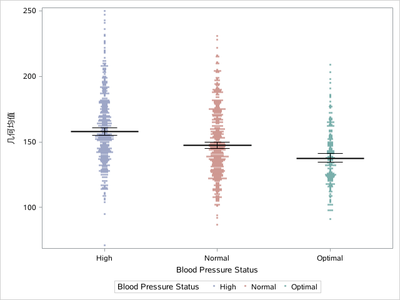## How to get line at geometric mean with 95% CI in scatter plot

I want to get a plot like this, with a line at the mean (or geometric mean) and bars for the 95% CI. I'm unsure how to get just the line though.``````data _Dummy;
do USUBJID = 1 to 9;
do ARMN= 1 to 5;
do ATPTN= 1, 8, 29, 36, 57, 64, 85, 180, 181, 187, 208, 304;
do PARAMN = 1 to 3; output; end;
output;
end; end; end;
data Dummy;
set _Dummy;
call streaminit(5797);
ATPT = 'Study Day '||strip(ATPTN);
if (ATPTN in (64 85 181) and ARMN = 3)|(ATPTN in (180 187 208 304) and ARMN ^= 3) then delete;

AVAL = rand("integer", 100, 300);	/*AVAL=Analysis Value*/
LAVAL = log(AVAL);					/*LAVAL=Log Analysis Value*/
run;

proc sgpanel data= Dummy noautolegend;
panelby ATPT / novarname onepanel columns= 3 headerattrs= (family= "times new roman" size= 9) headerbackcolor= "white" sort= data;
scatter x= PARAMN y= AVAL 	/ group= ARMN;
vbox AVAL					/ group= ARMN category= PARAMN nofill;
rowaxis label= "Worm Numbers";
format PARAMN paramn. ARMN armn.;
run;``````

1 ACCEPTED SOLUTION

Accepted SolutionsKsharp
Super User

## Re: How to get line at geometric mean with 95% CI in scatter plot

``````data have;
set sashelp.heart(obs=1000);
keep bp_status weight;
run;

proc sort data=have;by  bp_status;run;
ods select none;
ods output ConfLimits=ConfLimits ;
proc ttest data=have dist=lognormal;
by bp_status;
var weight;
run;
ods select all;

data want;
set have ConfLimits(keep=bp_status GeomMean LowerCLGeomMean UpperCLGeomMean);
run;
proc sgplot data=want;
scatter x=bp_status y=weight/jitter markerattrs=(symbol=circlefilled size=4) transparency=0.6 group=bp_Status;
scatter x=bp_Status y=GeomMean/yerrorlower=LowerCLGeomMean yerrorupper=UpperCLGeomMean
ERRORBARATTRS=(color=black) ERRORCAPSCALE=4 markerattrs=(size=0);
highlow x=bp_status high=GeomMean low=GeomMean/type=bar lineattrs=(thickness=2) barwidth=0.6;
run;``````5 REPLIES 5

## Re: How to get line at geometric mean with 95% CI in scatter plot

Please provide the formats paramn and armn.

--------------------------
The hash OUTPUT method will overwrite a SAS data set, but not append. That can be costly. Consider voting for Add a HASH object method which would append a hash object to an existing SAS data set

Would enabling PROC SORT to simultaneously output multiple datasets be useful? Then vote for
Allow PROC SORT to output multiple datasets

--------------------------

## Re: How to get line at geometric mean with 95% CI in scatter plot

Your code shows a box plot, but that is not part of your description or the figure you say you want.

Can you clarify, do you want a plot that shows (for each group)

1. jittered points that shows individual values

2. an estimate of central location, such as a mean

3. a confidence interval for the mean

## Re: How to get line at geometric mean with 95% CI in scatter plot

Yes to all. I’d like a jitter plot with individual measurements for each
group; a vertical line to represent the geometric mean; and 95% CI Of the
GM.

I’d pretty much like a graph like the example figure. I say GM instead of
arithmetic mean b/c of small sample size.

## Re: How to get line at geometric mean with 95% CI in scatter plot

In the picture, there is one class variable per panel. Do you want a panel that shows the ATPT values, and within each panel the groups are determined by the ARMN variable?  It looks like the PARMN variable is longitudinal (like different visits) and will be aggregated over?Ksharp
Super User

## Re: How to get line at geometric mean with 95% CI in scatter plot

``````data have;
set sashelp.heart(obs=1000);
keep bp_status weight;
run;

proc sort data=have;by  bp_status;run;
ods select none;
ods output ConfLimits=ConfLimits ;
proc ttest data=have dist=lognormal;
by bp_status;
var weight;
run;
ods select all;

data want;
set have ConfLimits(keep=bp_status GeomMean LowerCLGeomMean UpperCLGeomMean);
run;
proc sgplot data=want;
scatter x=bp_status y=weight/jitter markerattrs=(symbol=circlefilled size=4) transparency=0.6 group=bp_Status;
scatter x=bp_Status y=GeomMean/yerrorlower=LowerCLGeomMean yerrorupper=UpperCLGeomMean
ERRORBARATTRS=(color=black) ERRORCAPSCALE=4 markerattrs=(size=0);
highlow x=bp_status high=GeomMean low=GeomMean/type=bar lineattrs=(thickness=2) barwidth=0.6;
run;``````Discussion stats
• 5 replies
• 540 views
• 6 likes
• 4 in conversation